# gatherLabelData

Gather synchronized label data from ground truth

Since R2020a

## Syntax

``labelData = gatherLabelData(gTruth,signalNames,labelTypes)``
``[labelData,timestamps] = gatherLabelData(___)``
``[___] = gatherLabelData(___,Name=Value)``

## Description

````labelData = gatherLabelData(gTruth,signalNames,labelTypes)` returns synchronized label data gathered from multisignal ground truth data, `gTruth`. The function returns label data for the signals specified by `signalNames` and the label types specified by `labelTypes`.```

example

````[labelData,timestamps] = gatherLabelData(___)` additionally returns the signal timestamps associated with the gathered label data, using the arguments from the previous syntax.Use `timestamps` with the `writeFrames` function to write the associated signal frames from the `groundTruthMultisignal` objects to disk. Use these frames and the associated labels as training data for machine learning or deep learning models.```
````[___] = gatherLabelData(___,Name=Value)` specifies options using one or more name-value arguments in addition to any combination of arguments from previous syntaxes. For example, `Verbose=True` enables display to the workspace environment.```

## Examples

collapse all

Gather label data for a video signal and a lidar point cloud sequence signal from a `groundTruthMultisignal` object. Write the signal frames associated with that label data to disk and visualize the frames.

Add the point cloud sequence folder path to the MATLAB® search path. The video is already on the MATLAB search path.

```pcSeqDir = fullfile(toolboxdir('driving'),'drivingdata', ... 'lidarSequence'); addpath(pcSeqDir); ```

Load a `groundTruthMultisignal` object that contains label data for the video and the lidar point cloud sequence.

```data = load('MultisignalGTruth.mat'); gTruth = data.gTruth; ```

Specify the signals from which to gather label data.

```signalNames = ["video_01_city_c2s_fcw_10s" "lidarSequence"]; ```

The video contains rectangle labels, whereas the lidar point cloud sequence contains cuboid labels. Gather the rectangle labels from the video and the cuboid labels from the lidar point cloud sequence.

```labelTypes = [labelType.Rectangle labelType.Cuboid]; [labelData,timestamps] = gatherLabelData(gTruth,signalNames,labelTypes); ```

Display the first eight rows of label data from the two signals. Both signals contain data for the `Car` label. In the video, the `Car` label is drawn as a rectangle bounding box. In the lidar point cloud sequence, the `Car` label is drawn as a cuboid bounding box.

```videoLabelSample = head(labelData{1}) lidarLabelSample = head(labelData{2}) ```
```videoLabelSample = table Car _________________ {[299 213 42 33]} lidarLabelSample = table Car ____________________________________________________ {[17.7444 6.7386 3.3291 3.6109 3.2214 3.5583 0 0 0]} ```

Write signal frames associated with the gathered label data to temporary folder locations, with one folder per signal. Use the timestamps returned by the `gatherLabelData` function to indicate which signal frames to write.

```outputFolder = fullfile(tempdir,["videoFrames" "lidarFrames"]); fileNames = writeFrames(gTruth,signalNames,outputFolder,timestamps); ```
```Writing 2 frames from the following signals: * video_01_city_c2s_fcw_10s * lidarSequence ```

Load the written video signal frames by using an `imageDatastore` object. Load the associated rectangle label data by using a `boxLabelDatastore` object.

```imds = imageDatastore(fileNames{1}); blds = boxLabelDatastore(labelData{1}); ```

Load the written lidar signal frames by using a `fileDatastore` object. Load the associated cuboid label data by using a `boxLabelDatastore` object.

```fds = fileDatastore(fileNames{2},'ReadFcn',@pcread); clds = boxLabelDatastore(labelData{2}); ```

Visualize the written video frames by using a `vision.VideoPlayer` object. Visualize the written lidar frames by using a `pcplayer` object.

```videoPlayer = vision.VideoPlayer; ptCloud = preview(fds); ptCloudPlayer = pcplayer(ptCloud.XLimits,ptCloud.YLimits,ptCloud.ZLimits); while hasdata(imds) % Read video and lidar frames. I = read(imds); ptCloud = read(fds); % Visualize video and lidar frames. videoPlayer(I); view(ptCloudPlayer,ptCloud); end ```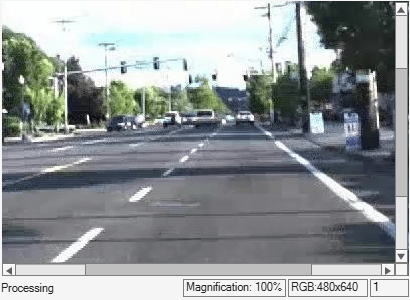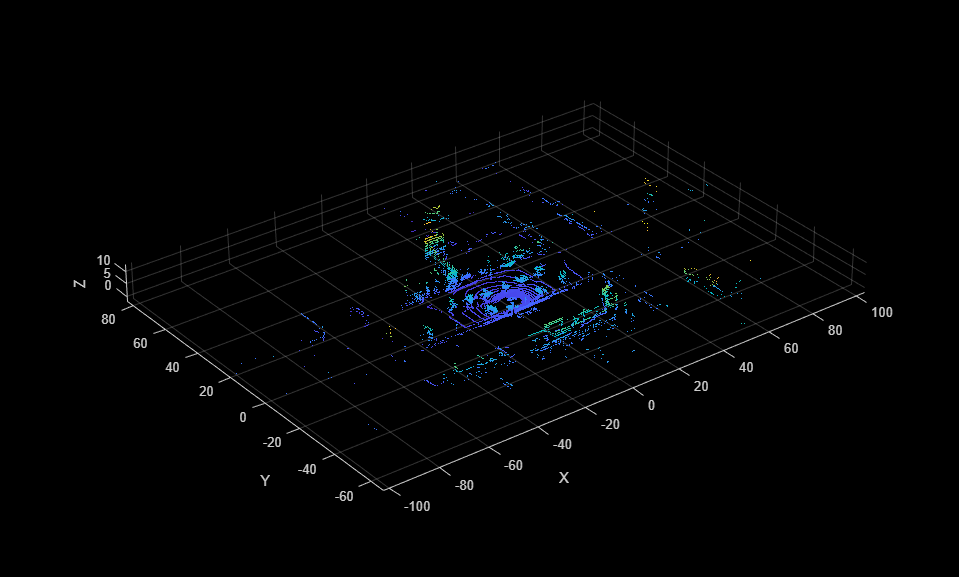Remove the path to the point cloud sequence folder.

```rmpath(pcSeqDir); ```

## Input Arguments

collapse all

Multisignal ground truth data, specified as a `groundTruthMultisignal` object or vector of `groundTruthMultisignal` objects.

Each `groundTruthMultisignal` object in `gTruth` must include all the signals specified in the `signalNames` input.

In addition, each object must include at least one marked label per gathered label definition. Suppose `gTruth` is a `groundTruthMultisignal` object containing label data for a single video signal named `video_front_camera`. The object contains marked rectangle region of interest (ROI) labels for the `car` label definition but not for the `truck` label definition. If you use this syntax to gather labels of type `Rectangle` from this object, then the `gatherLabelData` function returns an error.

`labelData = gatherLabelData(gTruth,"video_front_camera",labelType.Rectangle);`

Names of the signals from which to gather label data, specified as a character vector, string scalar, cell array of character vectors, or string vector. The signal names must be valid signal names stored in the input multisignal ground truth data, `gTruth`.

To obtain the signal names from a `groundTruthMultisignal` object, use this syntax, where `gTruth` is the variable name of the object:

`gTruth.DataSource.SignalName`

Example: `'video_01_city_c2s_fcw_10s'`

Example: `"video_01_city_c2s_fcw_10s"`

Example: `{'video_01_city_c2s_fcw_10s','lidarSequence'}`

Example: ```["video_01_city_c2s_fcw_10s" "lidarSequence"]```

Label types from which to gather label data, specified as a `labelType` enumeration scalar, `labelType` enumeration vector, or a cell array of `labelType` enumeration scalars and vectors. The `gatherLabelData` function gathers label data for each signal specified by input `signalNames` and each `groundTruthMultisignal` object specified by input `gTruth`. The number of elements in `labelTypes` must match the number of signals in `signalNames`.

#### Gather Label Data for Single Label Type per Signal

To gather label data for a single label type per signal, specify `labelTypes` as a `labelType` enumeration scalar or vector. Across all `groundTruthMultisignal` objects in `gTruth`, the `gatherLabelData` function gathers `labelTypes(n)` label data from `signalName(n)`, where `n` is the index of the label type and the corresponding signal name whose label data is to be gathered. Each returned table in the output `labelData` cell array contains data for only one label type per signal.

In this code sample, the `gatherLabelData` function gathers labels of type `Rectangle` from a video signal named `video_front_camera`. The function also gathers labels of type `Cuboid` from a lidar point cloud sequence signal stored in a folder named `lidarData`. The `gTruth` input contains the `groundTruthMultisignal` objects from which this data is to be gathered.

```labelData = gatherLabelData(gTruth, ... ["video_front_camera","lidarData"], ... [labelType.Rectangle,labelType.Cuboid];```

To gather label data for a single label type from separate signals, you must repeat the label type for each signal. In this code sample, the `gatherLabelData` function gathers labels of type `Rectangle` from the `video_left_camera` and `video_right_camera` video signals.

```labelData = gatherLabelData(gTruth, ... ["video_left_camera","video_right_camera"], ... [labelType.Rectangle,labelType.Rectangle];```

#### Gather Label Data for Multiple Label Types per Signal

To gather label data for multiple label types per signal, specify `labelTypes` as a cell array of `labelType` enumeration scalars and vectors. Across all `groundTruthMultisignal` objects in `gTruth`, the `gatherLabelData` function gathers `labelTypes{n}` label data from `signalName(n)`, where `n` is the index of the label types and the corresponding signal name whose label data is to be gathered. The function groups the data for these label types into one table per signal per `groundTruthMultisignal` object.

In this code sample, the `gatherLabelData` function gathers labels of type `Rectangle` and `Line` from the `video_front_camera` video signal. The function also gathers labels of type `Cuboid` from a lidar point cloud sequence signal stored in a folder named `lidarData`. The `gTruth` input contains the `groundTruthMultisignal` objects from which this data is to be gathered.

```labelData = gatherLabelData(gTruth, ... ["video_front_camera", ... "lidarData"], ... {[labelType.Rectangle labelType.Line], ... labelType.Cuboid};```

#### Valid Enumeration Types

You can specify one or more of these enumeration types.

• `labelType.Rectangle` — Rectangle ROI labels

• `labelType.RotatedRectangle` — Rotated Rectangle ROI labels

• `labelType.Cuboid` — Cuboid ROI labels (point clouds)

• `labelType.ProjectedCuboid` — Projected cuboid ROI labels (images and video data)

• `labelType.Line` — Line ROI labels

• `labelType.PixelLabel` — Pixel ROI labels

• `labelType.Polygon` — Pixel ROI labels

• `labelType.Scene` — Scene labels

To gather label data for scenes, you must specify `labelTypes` as the `labelType.Scene` enumeration scalar. You cannot specify any other label types with `labelType.Scene`.

### Name-Value Arguments

Specify optional pairs of arguments as `Name1=Value1,...,NameN=ValueN`, where `Name` is the argument name and `Value` is the corresponding value. Name-value arguments must appear after other arguments, but the order of the pairs does not matter.

Example: (`SamplingFactor`=`5`) sets the subsampling factor to `5` to drop every fifth frame.

Sample factor used to subsample label data, specified as a positive integer. A sample factor of `K` includes every `K`th signal frame. Increase the sample factor to drop redundant frames from signals with high sample rates, such as videos. To set the `SamplingFactor` with projected cuboid data, you must specify the `LabelData` name-value argument to `labelType.ProjectedCuboid`.

Use sampled data to reduce repeated data, such as a sequence of images with the same scene and labels. It can also help in reducing training time.

Group columns from label data, specified as `"LabelName"` or `"LabelType"`.

• `"LabelName"` — Groups the label data into columns by label definitions.

• `"LabelType"` — Groups the label data into columns by label type. You can use this option to gather label data while retaining the region-of-interest (ROI) stacking order determined by the label type.

Logical to indicate if attribute and sublabel data is returned in label data output, specified as a logical `false` (`0`) or `true` (`1`). A value of `false` indicates that only the position values that correspond to ROIs are collected. A value of `true` indicates that each table in `LabelData` contain structs that contain the position, attributes, and sublabels associated with each label.

## Output Arguments

collapse all

Label data, returned as an `M`-by-`N` cell array of tables, where:

• `M` is the number of `groundTruthMultisignal` objects in `gTruth`.

• When `labelTypes` contains ROI `labelType` enumerations, `N` is the number of signals in `signalNames` and the number of elements in `labelTypes`. In this case, `labelData{m,n}` contains a table of label data for the `n`th signal of `signalNames` that is in the `m`th `groundTruthMultisignal` object of `gTruth`. The table contains label data for only the label types in the `n`th position of `labelTypes`.

• When `labelTypes` contains only the `labelType.Scene` enumeration, `N` is equal to `1`. In this case, `labelData{m}` contains a table of scene label data across all signals in the `m`th `groundTruthMultisignal` object of `gTruth`.

For a given label data table, `tbl`, the table is of size `T`-by-`L`, where:

• `T` is the number of timestamps in the signal for which label data exists.

• `L` is the number of label definitions that are of the label types gathered for that signal.

• `tbl(t,l)` contains the label data gathered for the `l`th label at the `t`th timestamp.

If one of the signals has no label data at a timestamp, then the corresponding label data table does not include a row for that timestamp.

For each cell in the table, the format of the returned label data depends on the type of label.

Label TypeStorage Format for Labels at Each Timestamp
`labelType.Rectangle`

`M`-by-4 numeric matrix of the form `[x, y, w, h]`, where:

• `M` is the number of labels in the frame.

• `x` and `y` specify the upper-left corner of the rectangle.

• `w` specifies the width of the rectangle, which is its length along the x-axis.

• `h` specifies the height of the rectangle, which is its length along the y-axis.

`labelType.RotatedRectangle`

For one or more rotated rectangles, specify in spatial coordinates as an M-by-5 numeric matrix, where each row specifies a rotated rectangle of the form [xctr yctr w h yaw].

• M is the number of rotated rectangles.

• xctr and yctr specify the center of the rectangle.

• w specifies the width of the rectangle, which is its length along the x-axis before rotation.

• h specifies the height of the rectangle, which is its length along the y-axis before rotation.

• yaw specifies the rotation angle in degrees. The rotation is clockwise-positive around the center of the rectangle.

`labelType.Cuboid`

`M`-by-9 numeric matrix with rows of the form `[xctr, yctr, zctr, xlen, ylen, zlen, xrot, yrot, zrot]`, where:

• `M` is the number of labels in the frame.

• `xctr`, `yctr`, and `zctr` specify the center of the cuboid.

• `xlen`, `ylen`, and `zlen` specify the length of the cuboid along the x-axis, y-axis, and z-axis, respectively, before rotation has been applied.

• `xrot`, `yrot`, and `zrot` specify the rotation angles for the cuboid along the x-axis, y-axis, and z-axis, respectively. These angles are clockwise-positive when looking in the forward direction of their corresponding axes.

The figure shows how these values determine the position of a cuboid.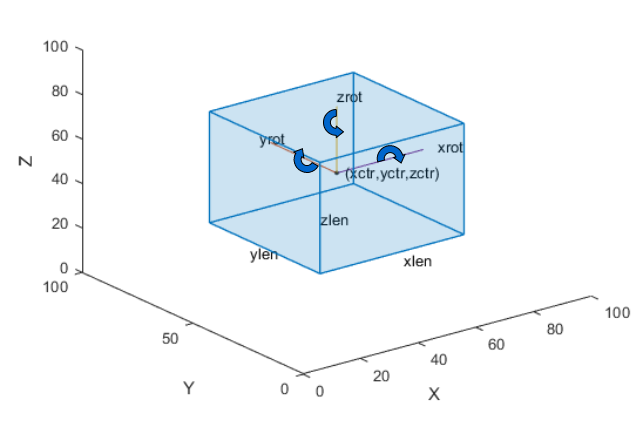`labelType.ProjectedCuboid`

`M`-by-8 vector of the form ```[x1, y1, w1, h1, x2, y2, w2, h2]```, where:

• `M` is the number of labels in the frame.

• `x1, y1` specifies the x,y coordinates for the upper-left location of the front-face of the projected cuboid

• `w1` specifies the width for the front-face of the projected cuboid.

• `h1` specifies the height for the front-face of the projected cuboid.

• `x2, y2` specifies the x,y coordinates for the upper-left location of the back-face of the projected cuboid.

• `w2` specifies the width for the back-face of the projected cuboid.

• `h2` specifies the height for the back-face of the projected cuboid.

The figure shows how these values determine the position of a cuboid.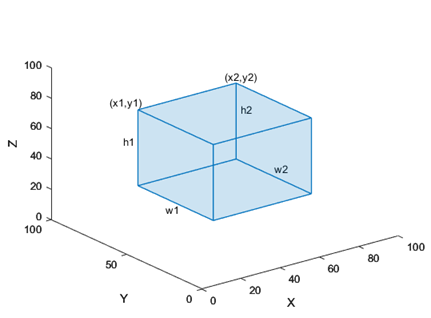`labelType.Line`

`M`-by-1 vector of cell arrays, where `M` is the number of labels in the frame. Each cell array contains an `N`-by-2 numeric matrix of the form `[x1 y1; x2 y2; ... ; xN yN]` for `N` points in the polyline.

`labelType.PixelLabel`

Label data for all pixel label definitions is stored in a single M-by-1 `PixelLabelData` column for M images or frames. Each element contains a filename for a pixel label image. A pixel label image describes the label or labels contained in the corresponding image. The labels can be described as a 1- or 3- channel label matrix. To use `PixelLabelData` with any of the labeler apps, you must use a single-channel label matrix, where the values are of type `uint8`. You can convert a 3-channel pixel label data matrix to a single-channel label matrix programmatically to use with the labeler apps.

`labelType.Polygon`

`M`-by-1 vector of cell arrays, where `M` is the number of labels. Each cell array contains an `N`-by-2 numeric matrix of the form `[x1 y1; x2 y2; ... ; xN yN]` for `N` points in the polygon.

`labelType.Scene`Logical `1` (`true`) if the scene label is applied. Otherwise logical `0` (`false`)

#### Label Data Format

Consider a cell array of label data gathered by using the `gatherLabelData` function. The function gathers labels from three `groundTruthMultisignal` objects with variable names `gTruth1`, `gTruth2`, and `gTruth3`.

• For a video signal named `video_front_camera`, the function gathers labels of type `Rectangle` and `Line`.

• For a lidar point cloud sequence signal stored in a folder named `lidarData`, the function gathers labels of type `Cuboid`.

This code shows the call to the `gatherLabelData` function.

```labelData = gatherLabelData([gTruth1 gTruth2 gTruth3], ... ["video_front_camera", ... "lidarData"], ... {[labelType.Rectangle labelType.Line], ... labelType.Cuboid};```
The `labelData` output is a 3-by-2 cell array of tables. Each row of the cell array contains label data for one of the `groundTruthMultisignal` objects. The first column contains the label data for the video signal, `video_front_camera`. The second column contains the label data for the point cloud sequence signal, `lidarData`. This figure shows the `labelData` cell array.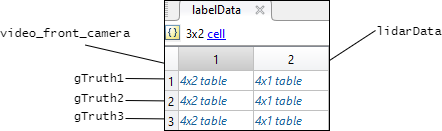This figure shows the label data table for the video signal in the third `groundTruthMultisignal` object. The `gatherLabelData` function gathered data for a `Rectangle` label named `car` and a `Line` label named `lane`. The table contains label data at four timestamps in the signal.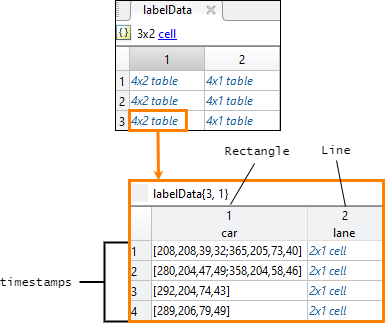This figure shows the label data table for the lidar signal in the third `groundTruthMultisignal` object. The `gatherLabelData` function gathered data for a `Cuboid` label, also named `car`. The `car` label appears in both signal types because it is marked as a `Rectangle` label for video signals and a `Cuboid` label for lidar signals. The table contains label data at four timestamps in the signal.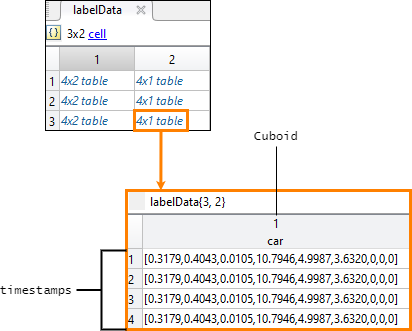Signal timestamps, returned as an `M`-by-`N` cell array of `duration` vectors, where:

• `M` is the number of `groundTruthMultisignal` objects in `gTruth`.

• `N` is the number of signals in `signalNames`.

• `labelData{m,n}` contains the timestamps for the `n`th signal of `signalNames` that is in the `m`th `groundTruthMultisignal` object of `gTruth`.

If you gather label data from multiple signals, the signal timestamps are synchronized to the timestamps of the first signal specified by `signalNames`.

## Version History

Introduced in R2020a